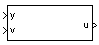# 1D Controller Blend: u=(1-L).K1.y+L.K2.y

Implement 1-D vector of state-space controllers by linear interpolation of their outputs

•Libraries:
Aerospace Blockset / GNC / Control

## Description

The 1D Controller Blend u=(1-L).K1.y+L.K2.y block implements an array of state-space controller designs. The model runs the controllers in parallel and interpolates their outputs according to the current flight condition or operating point. The advantage of this implementation approach is that the state-space matrices A, B, C, and D for the individual controller designs do not need to vary smoothly from one design point to the next. The output from this block is the actuator demand, which you can input to an actuator block.

## Limitations

This block requires the Control System Toolbox™ license.

## Ports

### Input

expand all

Aircraft measurements, specified as a vector.

Data Types: `double`

Scheduling variable, specified as a vector, that conforms to the dimensions of the state-space matrices.

Data Types: `double`

### Output

expand all

Actuator demands, specified as a vector.

Data Types: `double`

## Parameters

expand all

A-matrix of the state-space implementation, specified as a array. In the case of 1-D blending, the A-matrix should have three dimensions, the last one corresponding to scheduling variable v. For example, if the A-matrix corresponding to the first entry of v is the identity matrix, then `A(:,:,1) = [1 0;0 1];`.

#### Programmatic Use

 Block Parameter: `A` Type: character vector Values: vector Default: `'A1'`

B-matrix of the state-space implementation, specified as a array. In the case of 1-D scheduling, the B-matrix should have three dimensions, the last one corresponding to the scheduling variable v. For example, if the B-matrix corresponding to the first entry of v is the identity matrix, then `B(:,:,1) = [1 0;0 1];`.

#### Programmatic Use

 Block Parameter: `B` Type: character vector Values: vector Default: `'B1'`

C-matrix of the state-space implementation, specified as a array. In the case of 1-D scheduling, the C-matrix should have three dimensions, the last one corresponding to the scheduling variable v. For example, if the C-matrix corresponding to the first entry of v is the identity matrix, then `C(:,:,1) = [1 0;0 1];`.

#### Programmatic Use

 Block Parameter: `C` Type: character vector Values: vector Default: `'C1'`

D-matrix of the state-space implementation, specified as a array. In the case of 1-D scheduling, the D-matrix should have three dimensions, the last one corresponding to the scheduling variable v. For example, if the D-matrix corresponding to the first entry of v is the identity matrix, then `D(:,:,1) = [1 0;0 1];`.

#### Programmatic Use

 Block Parameter: `D` Type: character vector Values: vector Default: `'D1'`

Breakpoints for the scheduling variable, specified as a vector. The length of v must be same as the size of the third dimension of A, B, C, and D.

#### Programmatic Use

 Block Parameter: `breakpoints_v` Type: character vector Values: vector Default: ```'[1 1.5 2]'```

Initial states for the controller, such as initial values for the state vector, x, specified as a vector. The length must equal the size of the first dimension of A.

#### Programmatic Use

 Block Parameter: `x_initial` Type: character vector Values: vector Default: `'0'`

Poles of observer, specified as a vector. For incoming controllers, the block uses an observer-like structure to ensure that the controller output tracks the current block output, u. The number of poles must equal the dimension of the A-matrix. Poles that are too fast result in sensor noise propagation; poles that are too slow result in the failure of the controller output to track u.

#### Programmatic Use

 Block Parameter: `vec_w` Type: character vector Values: vector Default: ```'[-5 -2]'```

## Algorithms

The block implements

`$\begin{array}{l}{\stackrel{˙}{x}}_{1}={A}_{1}{x}_{1}+{B}_{1}y\\ {u}_{1}={C}_{1}{x}_{1}+{D}_{1}y\\ {\stackrel{˙}{x}}_{2}={A}_{2}{x}_{2}+{B}_{2}y\\ {u}_{2}={C}_{2}{x}_{2}+{D}_{2}y\\ u=\left(1-\lambda \right){u}_{1}+\lambda {u}_{2}\\ \\ \lambda =\left\{\begin{array}{ccc}0& & v<{v}_{\mathrm{min}}\\ \frac{v-{v}_{\mathrm{min}}}{{v}_{\mathrm{max}}-{v}_{\mathrm{min}}}& & {v}_{\mathrm{min}}\le v\le {v}_{\mathrm{max}}\\ 1& & v>{v}_{\mathrm{max}}\end{array}\\ \end{array}$`

For example, suppose two controllers are designed at two operating points v=vmin and v=vmax. For longer arrays of design points, the block only implements nearest neighbor designs. At any given instant in time, the block updates three controller designs, reducing computational requirements.

As the value of the scheduling parameter varies and the index of the controllers that need to be run changes, the block initializes the states of the oncoming controller using the self-conditioned form as defined for the Self-Conditioned [A,B,C,D] block.

 Hyde, R. A., "H-infinity Aerospace Control Design — A VSTOL Flight Application." , Advances in Industrial Control Series, Springer Verlag, 1995.